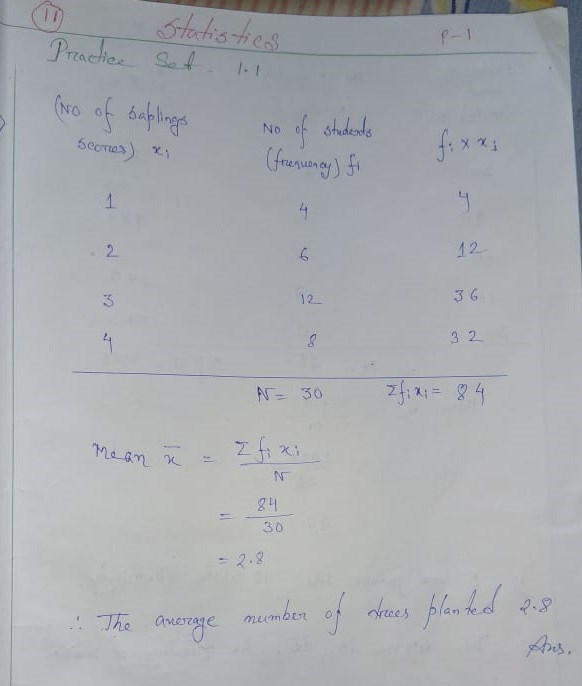# Maharashtra Board Class 8 Math Statistics Chapter Practice Set 1 Solution

Maha Board (MSBHSE) Class 8 Math (गणित) Statistics Practice Set 11.1 Solution (Page No. 69) in our website. Here candidates of Marathi Medium & English Medium of Maharashtra State can follow this to get Most Easy Solution of Maharashtra Board Class 8 Chapter 11: Statistics (Practice Set 11.1).

### Maharashtra Board Class 8 Math Practice Set 11.1 Solution | BalBharati Class 8 Mathematics Chapter 11 Solution:

 Subject Mathematics (गणित) Class 8 (८) Chapter 11 (११) Practice Set 11.1 (११. १)

### Practice Set 11.1 Solution (Page No. 69)

(1.) The following table shows the number of saplings planted by 30 students. Fill in the boxes and find the average number of saplings planted by each student.

 No. of saplings (Scores) x1 No. of students (frequency) f1 F1 x x1 1 4 4 2 6 ____ 3 12 ____ 4 8 _____ N = ____ Σf1x1 = ____

SOLUTION:(2.) The following table shows the electricity (in units) used by 25 families of Eklara village in a month of May. Complete the table and answer the following questions.

(1) How many families use 45 units electricity ?

(2) State the score, the frequency of which is 5.

3) Find N, and Σf1x1

(4) Find the mean of electricity used by each family in the month of May.

Solution:(3.) The number of members in the 40 families in Bhilar are as follows:

1, 6, 5, 4, 3, 2, 7, 2, 3, 4, 5, 6, 4, 6, 2, 3, 2, 1, 4, 5, 6, 7, 3, 4, 5, 2, 4, 3, 2, 3, 5, 5, 4, 6, 2, 3, 5, 6, 4, 2. Prepare a frequency table and find the mean of members of 40 families.

Solution:(4.) The number of Science and Mathematics projects submitted by Model high school, Nandpur in last 20 years at the state level science exibition is : 2, 3, 4, 1, 2, 3, 1, 5, 4, 2, 3, 1, 3, 5, 4, 3, 2, 2, 3, 2. Prepare a frequency table and find the mean of the data.

Solution:Latest Edition BalBharati Math Solution for Standard 8th Chapter 11.1. Any Kinds of problem to understand, Feel free to comment us below. Thank You.

Updated: February 20, 2020 — 11:42 am